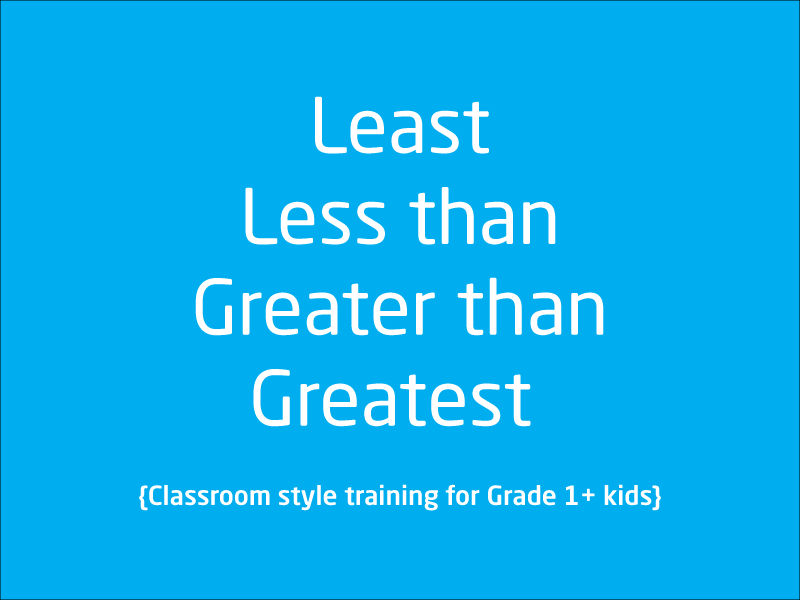# Free video lessons for Foundation to Grade 8 students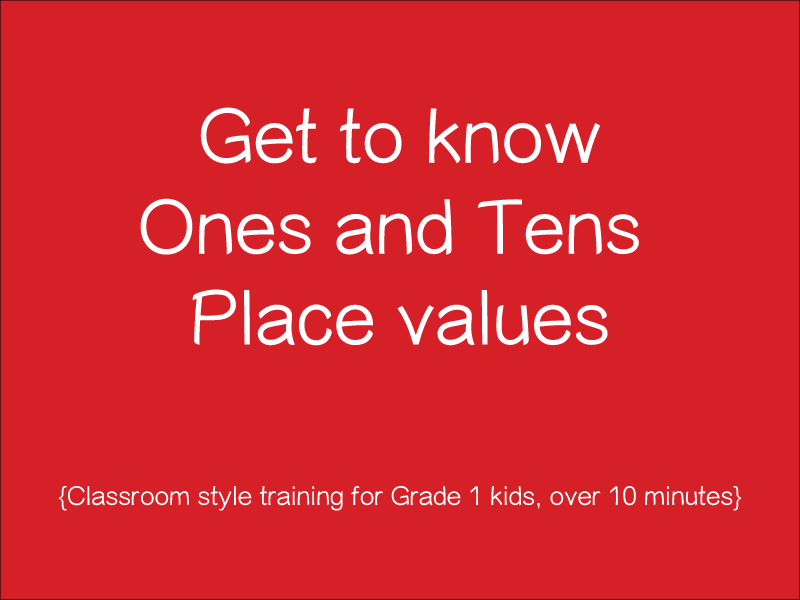### Ones and Tens Video Lesson

A classroom style training for kids who wish to get started with ones and tens place values. Tutor in this lesson provides with several examples. Please remember that this is a cla...

1 videos
Basic difficulty
0 0 0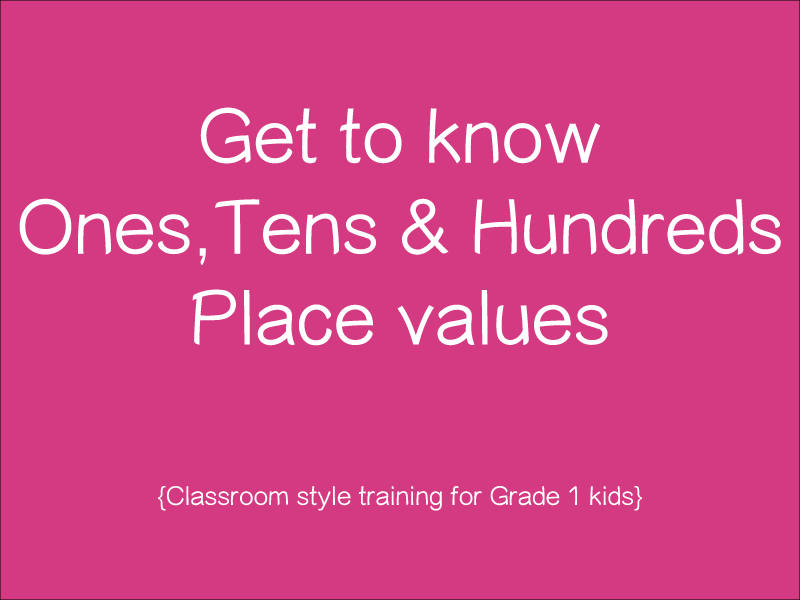### Ones, Tens and Hundreds

In this lesson, tutor will talk about the Ones, tens and hundreds place values. This is a classroom-style lesson and does not use cartoons and other props. There are few examples d...

1 videos
Basic difficulty
0 0 0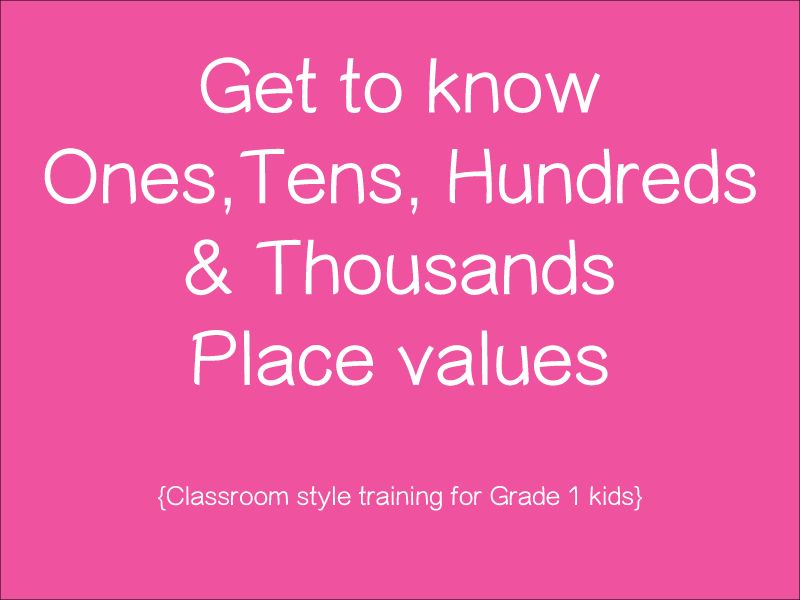### Place values

This is a classroom-style lesson that touch base on Ones, Tens, Hundreds and Thousands place values. It is recommended to watch previous lessons on Place values. If you see any iss...

1 videos
Basic difficulty
0 0 0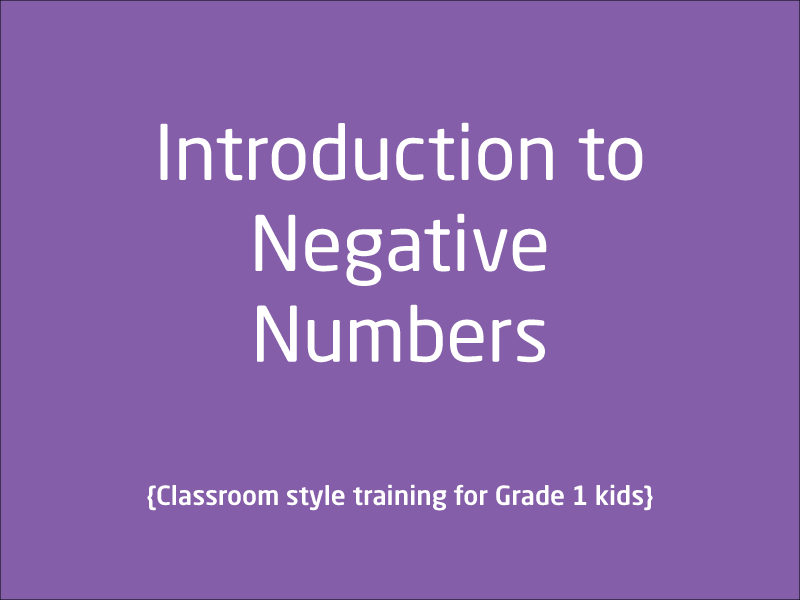### Negative Numbers

This is a classroom style lesson that gives introduction to Negative numbers. Thing to note is that, this is just an introduction fo negative numbers to get kids start to thin...

1 videos
Basic difficulty
0 0 0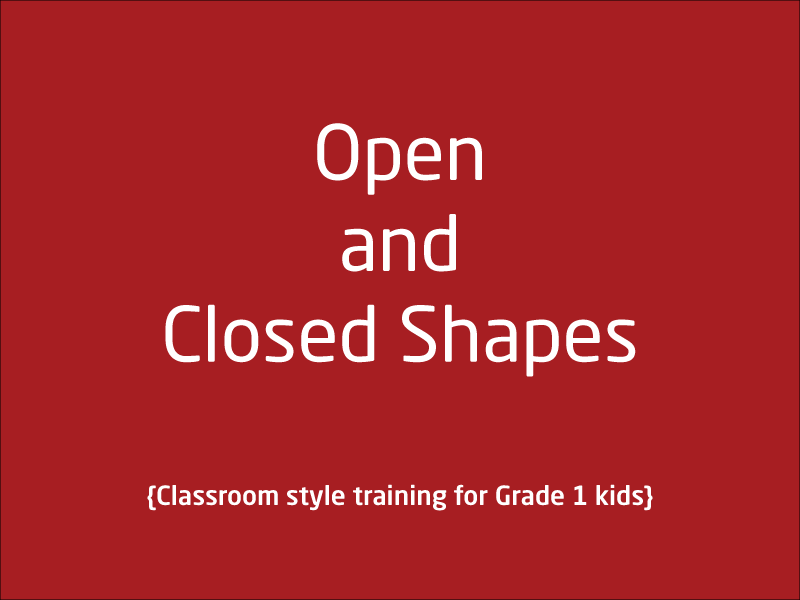### Open and Closed Shapes

In this lesson, kids will get to know about open and closed shapes. This is a classroom-style lesson with live use of whiteboard. Let us know if this lesson was helpful, we recomme...

1 videos
Basic difficulty
0 0 0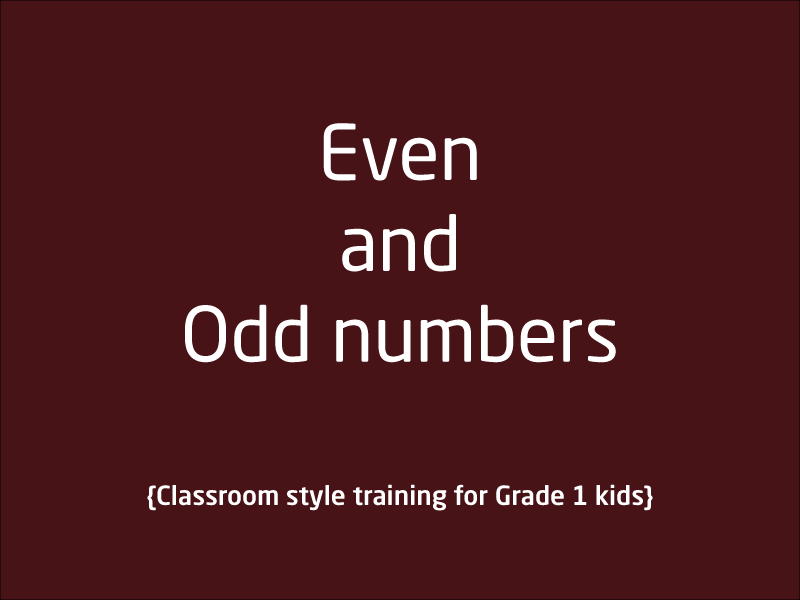### Even and Odd Numbers

What are even numbers? What are odd numbers? This lesson just explains the concepts of Odd and Even numbers. Lesson itself does not tell if Zero is a even or Odd number but as an e...

1 videos
Basic difficulty
0 0 0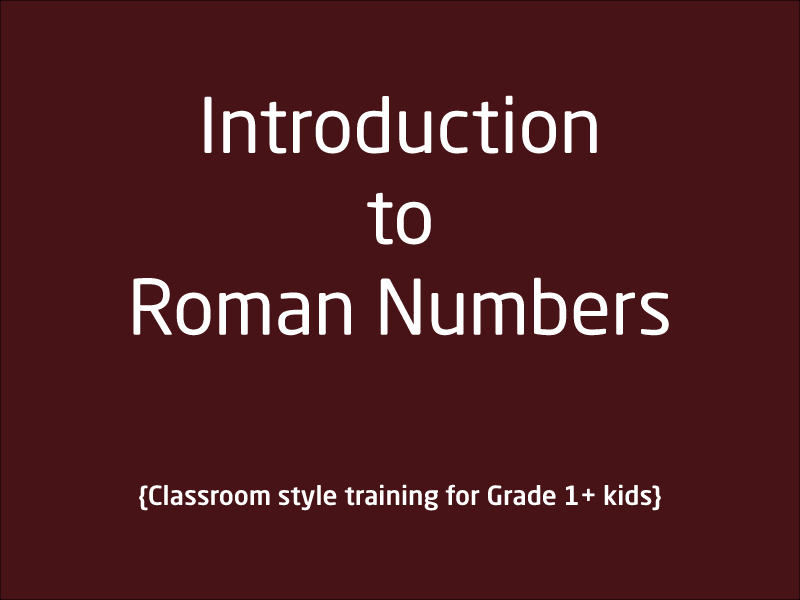### Roman Numbers

This lesson talks about the roman representation of whole/decimal numbers in Maths. Roman numerals are still used in many places. This is a classroom-style lesson and tutor use whi...

1 videos
Intermediate difficulty
0 0 0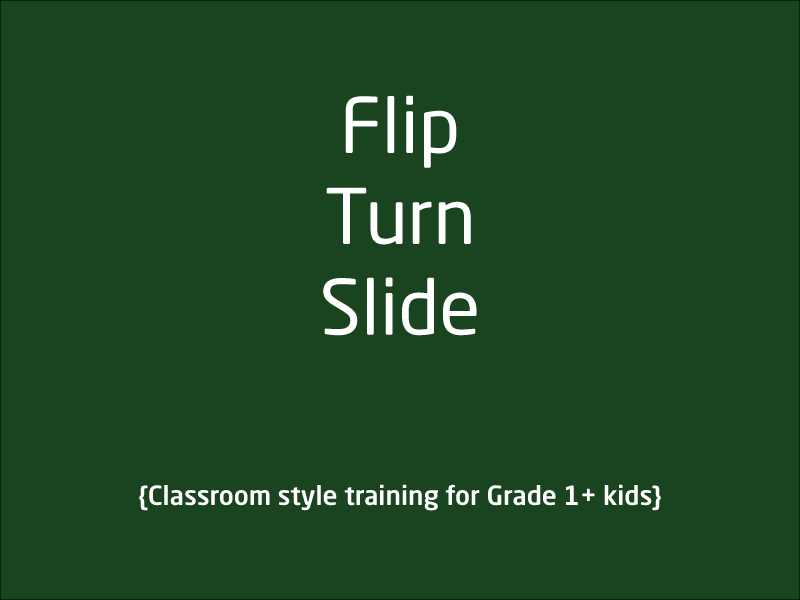### Flip Turn and Slide

In this lesson, tutor use interactive approach to explain fundamentals behind flip, turn and slide. This is a classroom-style lesson and tutor use whiteboard and pen excessively in...

1 videos
Basic difficulty
0 0 0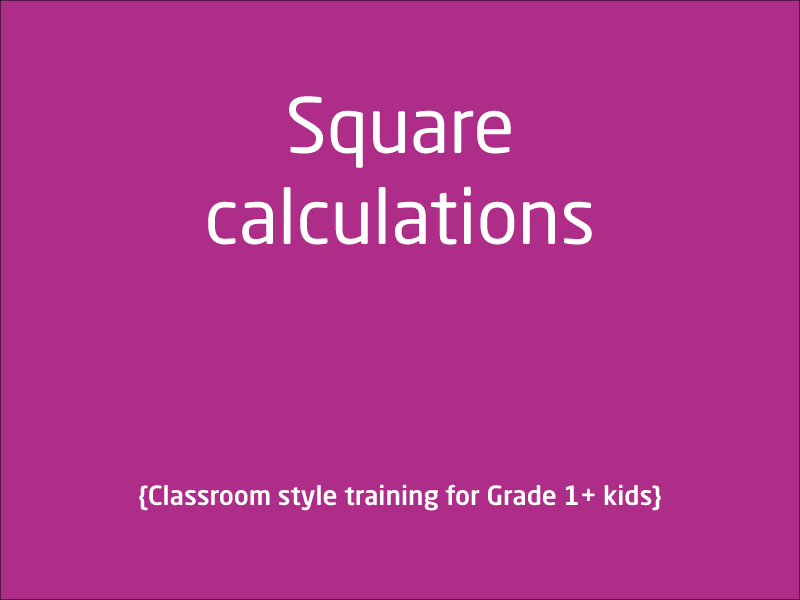### What is Square in Maths?

This lesson is for Grade 1+ kids or kids aged 6 years plus. In this lesson, tutor explain what exactly is a square and how exactly do we calculate the square of a number. Tutor pro...

1 videos
Basic difficulty